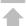SQL引擎

FileSystem引擎

S3引擎

SQL语法

SAC 管控中心

Web服务

# \$multiply

## 语法

`{ <字段名>: { \$multiply: <值> } }`

## 示例

`> db.foo.bar.insert( { "a": 3 } )`

• 作为选择符使用，返回字段“a”乘以10的结果：

```> db.foo.bar.find( {}, { "a": { "\$multiply": 10 } } )
{
"_id": {
"\$oid": "58256a7b2b4c38286d000020"
},
"a": 30
}
Return 1 row(s).```
• 与匹配符配合使用，匹配字段“a”乘以10之积为30的记录：

```> db.foo.bar.find( { "a": { "\$multiply": 10, "\$et": 30 } } )
{
"_id": {
"\$oid": "58256a7b2b4c38286d000020"
},
"a": 3
}
Return 1 row(s).```